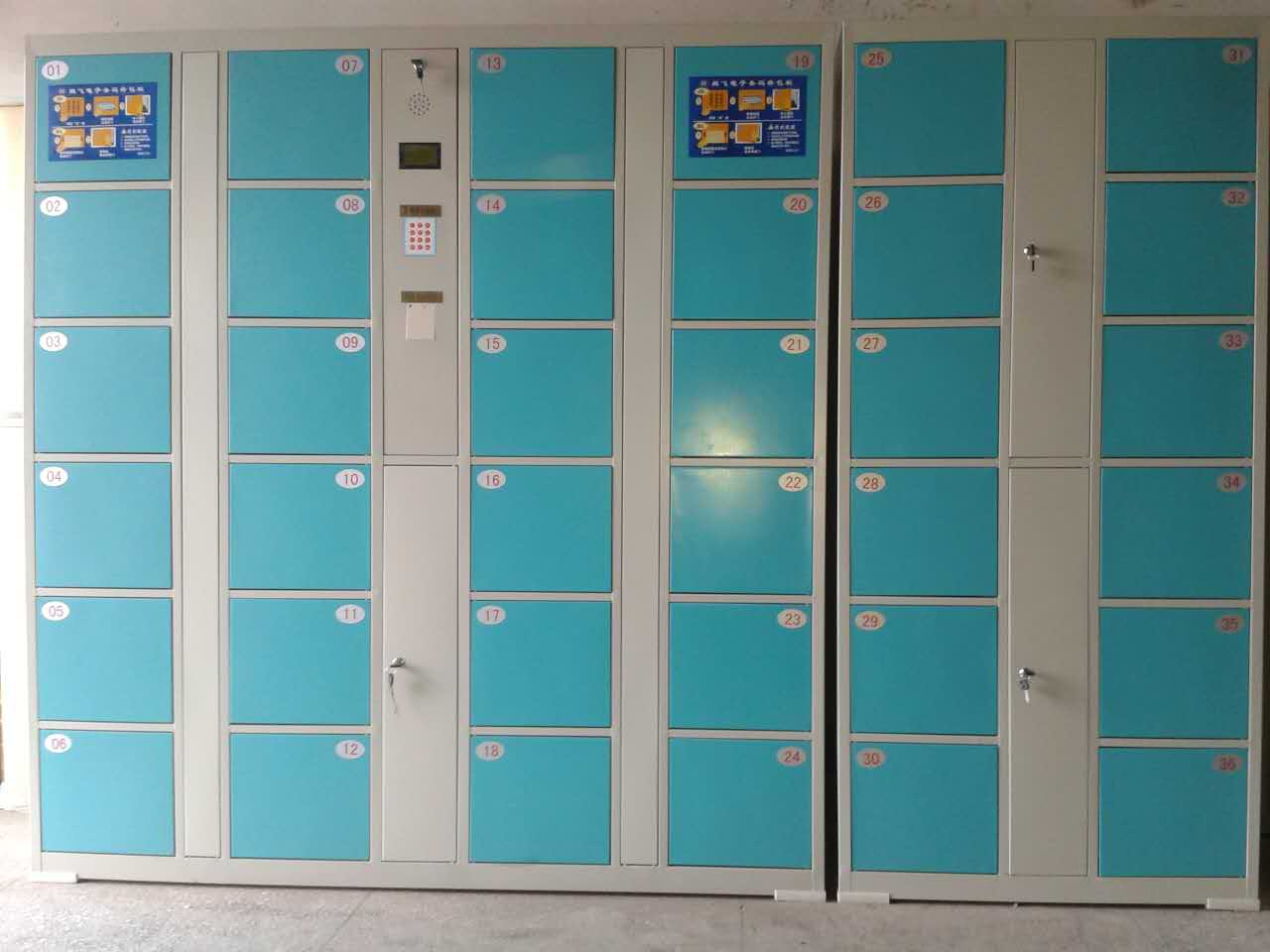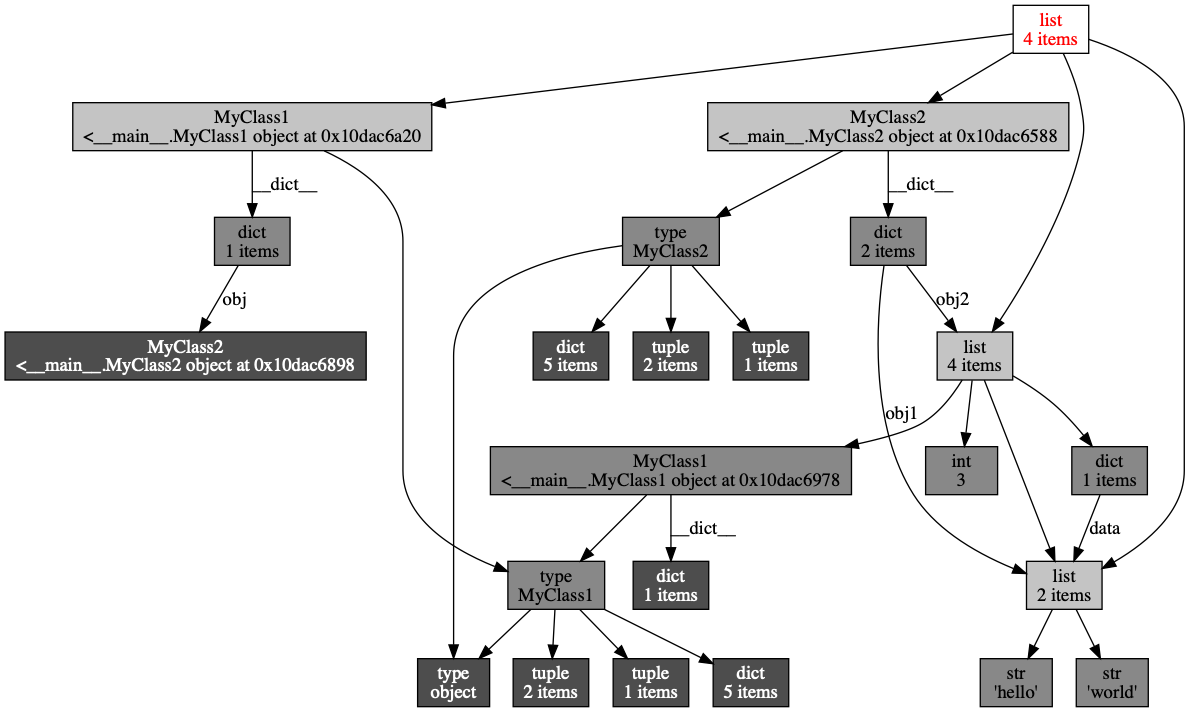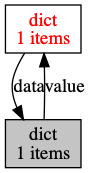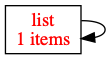## Python深度探索（1）：内存管理机制

1. 重复使用内存空间

n = 1 # 将1赋给变量n

Python作为动态语言，采用了引用与对象分离的策略，这也使得任何引用都可以指向任何对象，而且可以动态改变引用指向的对象类型，也就是说，可以将一个指向int类型的对象的引用重新指向bool类型的对象。所以可以将Python语言的对象模型看做是超市里的储物柜（这里只是用储物柜作为内存模型的比喻，不要与超市储物柜实际的操作进行比较）。x = 10
y = 10
z = 10

x、y和z三个变量的值都是10，这个10就相当于要保存在小柜子中的物品。x、y和z相当于3把钥匙。而3个变量中的值都是10，所以被认为是同一个值（物品），因此，就只需要动用一个小柜子保存10，而3个变量都会指向这个小柜子（由于计算机中值具有无限可复制性，所以只要有一个物品，就可以无限复制，所以不必考虑现实中将小柜子中的东西拿走了就为空的情况）。所以其实x、y和z这3个变量指向了同一个内存地址（相当于小柜子的序号）。可以用id函数验证这3个变量的内存地址是否相同，代码如下：

print(id(x))
print(id(y))
print(id(z))

4470531424
4470531424
4470531424

print(hex(id(x)))
print(hex(id(y)))
print(hex(id(z)))

0x10a76e560
0x10a76e560
0x10a76e560

print(x is y is z) # 输出结果：True

class MyClass:
pass
a = []
b = []
c = MyClass()
d = MyClass()
t1 = (1,2,3)
t2 = (1,2,3)
print(a is b) # False 元素个数和类型相同的列表不会使用同一个内存空间（小柜子）
print(c is d) # False MyClass类的不同实例不会使用同一个内存空间（小柜子）
print(t1 is t2) # True 元素个数和类型相同的元组会使用同一个内存空间（小柜子）

2. 引用计数器

from sys import getrefcount

a = [1, 2, 3]
print(getrefcount(a)) # 输出2

b = a
print(getrefcount(b)) # 输出3
print(getrefcount(a)) # 输出3

x = 1
print(getrefcount(x)) #输出1640
y = 1
print(getrefcount(x)) # 输出1641
print(getrefcount(y)) # 输出1641

3. 对象引用

from sys import getrefcount

# 类的构造方法传入另外一个对象的引用
class MyClass(object):
def __init__(self, other_obj):
self.other_obj = other_obj # 这里的other_obj与后面的data指向了同一块内存地址

data = {'name':'Bill','Age':30}
print(getrefcount(data)) # 输出2
my = MyClass(data)
print(id(my.other_obj)) # 输出4364264288
print(id(data)) #输出4364264288

print(getrefcount(data)) # 输出3

a = 1
b = 1

4. 循环引用与拓扑图

class MyClass1:
def __init__(self, obj):
self.obj = obj

class MyClass2:
def __init__(self,obj1,obj2):
self.obj1 = obj1
self.obj2 = obj2

data1 = ['hello', 'world']
data2 = [data1, MyClass1(data1),3,dict(data = data1)]
data3 = [data1,data2,MyClass2(data1,data2),MyClass1(MyClass2(data1,data2))]

import objgraph
objgraph.show_refs([data3], filename='对象引用关系.png')

show_refs函数会在当前目录下生成一个”对象引用关系.png“的图像文件，如下图所示。import objgraph
from sys import getrefcount
a = {}
b = {'data':a}
a['value'] = b
objgraph.show_refs([b], filename='循环引用1.png')a = {}
a['value'] = a
a = []
a.append(a)
print(getrefcount(a))
objgraph.show_refs([a], filename='循环引用2.png')5. 减少引用计数的两种方法

（1）用del删除某一个引用
（2）将变量指向另外一个引用，或设置为None，也就是引用重定向。

（1）用del删除某一个引用

del语句可以删除一个变量对某一个块内存空间的引用，也可以删除集合对象中的某个item，代码如下：

from sys import getrefcount

person = {'name':'Bill','age':40}
person1 = person
print(getrefcount(person1)) # 输出3

del person # 删除person对字典的引用
print(getrefcount(person1)) # 由于引用少了一个，所以输出为2
# print(person) # 抛出异常 # 被删除的变量相当于重来没定义过，所以这条语句会抛出异常

del person1['age'] # 删除字典中key为age的值对
print(person1)

（2）引用重定向
from sys import getrefcount

value1 = [1,2,3,4]
value2 = value1
value3 = value2
print(getrefcount(value2)) # 输出4
value1 = 20
print(getrefcount(value2)) # 输出3，因为value1重新指向了20
value3 = None
print(getrefcount(value2)) # 输出2，因为value3被设置为None，也就是不指向任何内存空间，相当于空指针

6. 垃圾回收import gc
print(gc.get_threshold())

(700, 10, 10)

gc.collect()

7. 变量不用了要设置为None

person = {'Name':'Bill'}
value = [1,2,3]
del person
value = None

8. 解决循环引用的回收问题

import objgraph
from sys import getrefcount
a = {}
b = {'data':a}
a['value'] = b
del a
del b

9. 分代回收import gc gc.set_threshold(600, 5, 6)

1. 引用计数策略（为0时释放）
2. 循环引用策略（将相关引用计数器多减1）
3. 分代策略（解决了GC的效率问题）posted on 2019-12-30 08:44  银河使者  阅读(656)  评论(0编辑  收藏  举报